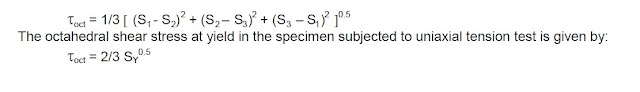# 15 Important Questions Related to Pipe Under Stress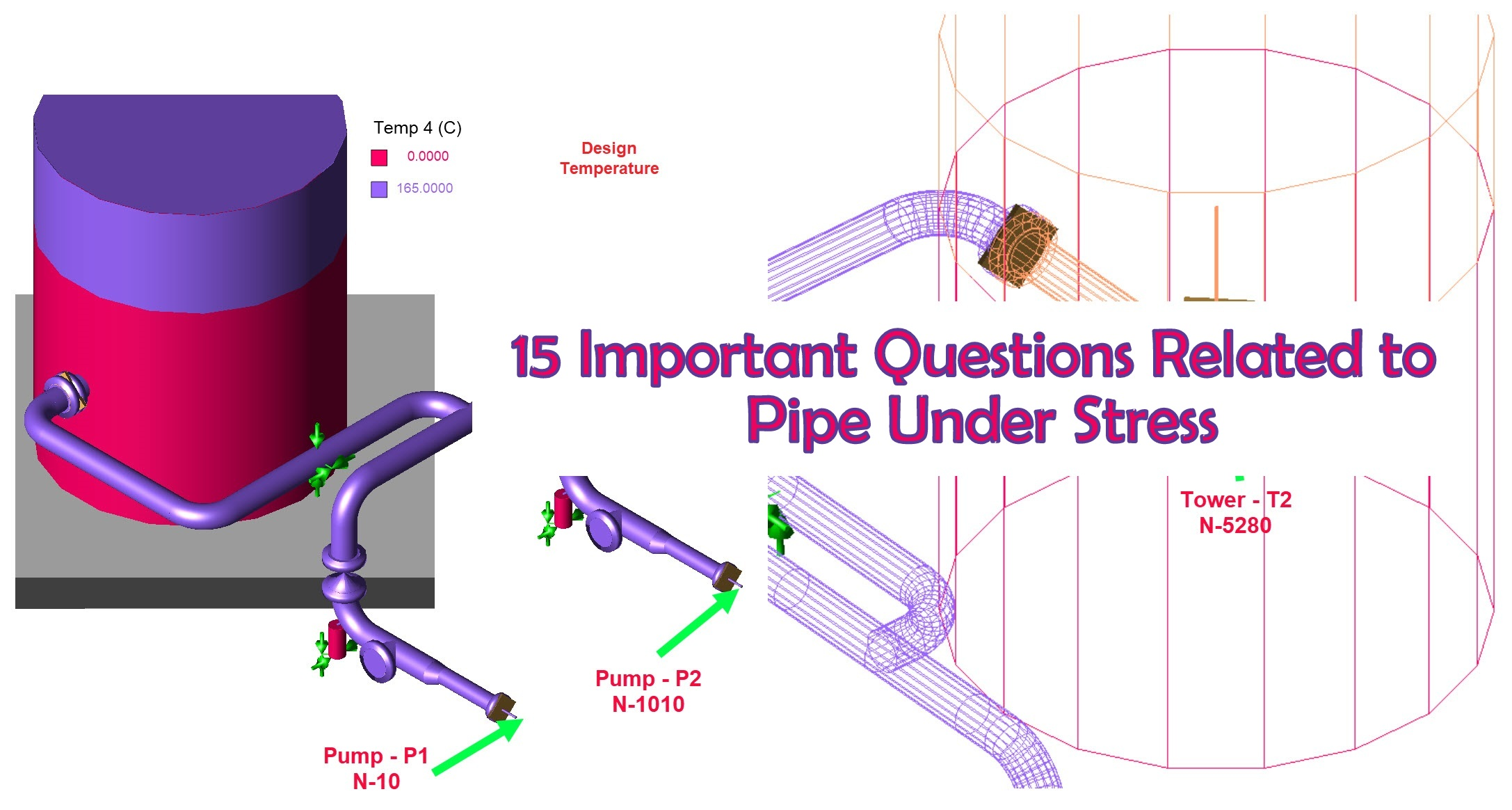These are typically steady or sustained types of loads. These loads have their origin in some force acting on the pipe causing tension, compression, torsion etc leading to normal and shear stress. Primary loads are not self limiting. Some of the primary loads are as:-
A. Internal fluid pressure
B. External pressure.
C. Gravitational forces acting on the pipe such as weight of the pipe & fluid.
D. Forces due to relief or blow down.
E. Pressure waves generated due to water hammer effects.

2. What do you mean by self limiting?

It means that the stresses continue to exist as long as long the load persists and deformation does not stop because the system has deformed into a no-stress condition but because strain hardening has come into play.

Secondary loads are caused by displacement of some kind. Some of the secondary loads are as:-
A. Force on piping due to tank settlement.
B. Vessel nozzle moving up due to expansion of vessel.
C. Pipe expansion or contraction.
D. Vibration due to rotational equipment.

4. What is the most used choice of co-ordinate system for defining the stresses?

In a pipe subjected to internal pressure or any other load, the most used choice of co-ordinate system is as:
A. Axial or Longitudinal direction.
B. Circumferential or Hoope’s direction.
The stresses in the pipe wall are expressed as axial(SL), Hoope’s (SH) and Radial (SR).These stresses which stretch or compress a grain/ crystal are called normal stress because they are normal to the surface of the crystal.

5. What do you mean by Hoop Stresses and how do you calculate it?

Stresses which are generated circumferentially due to the action of Internal pressure of pipe are called Hoop Stress. It is calculated by; -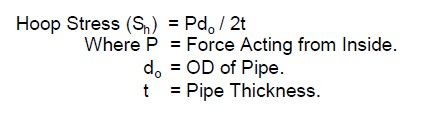6. How does Hoop Stress affect the system?

As per membrane theory for pressure design of cylinders, as long as hoop stress is less than yield stress of Moc, the design is safe. Hoop stress induced by thermal pressure is twice the axial stress (SL). This is widely used for pressure thickness calculation for pressure vessel.

7. What are the other stresses against which the design of piping is safe guarded?
Principal stress. B. Shear stress.
Apart from the stress which is normal to the surface of the crystal as mentioned in question No. 4, the grains would have been oriented in the pipe wall in all possible orientations. The above stresses (Axial, Circumferential and Radial stress) have stress component in direction normal to faces of randomly oriented crystal. Each crystal thus faces normal stresses. One of these orientations must be such that it maximizes one of the normal stresses. Normal stresses for such orientation (maximum normal stress orientation) are called principal stresses and are designated as S1 (maximum), S2 and S3 (minimum). Principal stresses are way of defining the worst case scenario as far as the normal stresses are concerned.

In addition to the normal stresses, a grain can be subjected to shear stresses as well. These stress act parallel to the crystal surface. The shear stresses occur if the pipe is subjected to torsion, bending etc. Just as there is an orientation for which normal stresses are maximum, there is an orientation which maximizes shear stress. The maximum shear stress in a 3-D state of stress can be shown to
be as:-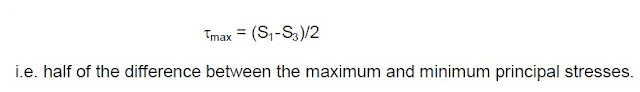8. What does the solid mechanics states regarding the Normal stresses?
Solid mechanic states that the sum of the three normal stresses for all orientation is always the same
for any given external load as:-

SL+ SH + SR = S1 + S2 + S3

9. Which component of normal stress is considered negligible?

In most pipe design cases, the radial component of normal stresses (SR) is negligible as compare to the other two component (SH and SL).

10. How the two principal stresses and maximum shear stress are calculated?

Use of Mohr’s circle allows calculating the two principal stresses and maximum shear stress as:-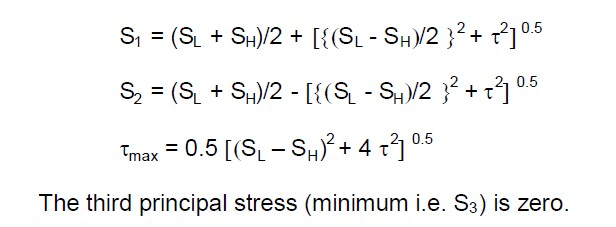11. What are the potential loads faced by pipes during normal operation? Write their relationship to the stresses developed?

The potential loads faced by a pipe are:-

12. What are the Theories of failure?

Important theories in common use are:-
A. Maximum Stress Theory or Rankine Theory.
B. Maximum Shear Theory or Tresca Theory.
C. Octahedral Shear Theory or Von Mises Theory.

13. What is Maximum stress theory?
According to this theory, failure occurs when the maximum principal stress in a system (S1) is greater than the maximum tensile principal stress at yield in a specimen subjected to uniaxial test. In uniaxial test, the applied load give rise to axial stress (SL) only and Hoope’s stress (SH) & Radial stress (SR) as well as the shear stress are absent. In a specimen under uniaxial tension test at yield the following
holds.

SL = SY, SH = 0, SR = 0
S1 = SY, S2 = 0, and S3= 0

The maximum tensile principle stress at yield is thus equal to the conventionally reported yield stress (load at yield / cross- sectional area of specimen).

The Rankine theory thus says that the failure occurs when the maximum principal stress in a system (S1) is more than the yield stress of the material (SY).

14. What is Maximum Shear Theory?

According to this theory the failure occurs when the maximum shear stress (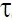max) is greater than the maximum shear stress at yield in a specimen subjected to uniaxial tension test.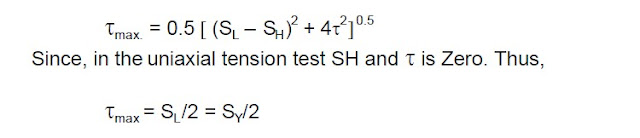The Tresca Theory thus says that the failure occurs when the maximum shear stress in a system (max) is more than half the yield stress of the material.

15. What is Octahedral Shear Theory?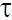oct.) is greater than the octahedral shear stress at the yield in a specimen subjected to uniaxial tension